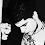## Thursday, August 11, 2005

### Sudoku Revisited

Robin Houston writes
Although I'm not a complexity theorist, I very much enjoy reading your weblog. I also enjoy solving the Sudoku puzzles published in the British press, so it was doubly nice to see your May 25 post about the complexity of Sudoku!

As far as I can tell, it follows from Yato's work that the problem:

1. Given a partially completed grid, find a valid completion if there is one; otherwise report that there isn't one.

is solvable in polynomial time iff P=NP.

That's interesting of course, and it's a problem that faces those who set the puzzles; but the problem that we solvers are faced with is not quite (1). It's:

2. Given a partially completed grid that has a unique valid completion, find that completion.
Can anything be said about problem (2)? If there were a polynomial- time algorithm for (2), would it follow that P=NP? If not, would there be any other significant consequences for complexity theory?
Good question. Since Yato's reductions preserve solutions the problem is equivalent to finding a satisfying assignment of a Boolean formula that has exactly one satisfying assignment (Unique SAT).

We don't know if Unique SAT is NP-complete in the traditional sense. However Valiant and Vazirani have a nice paper that shows how to randomly reduce SAT to Unique SAT. Putting it together we get the following equivalence:

• Given a partially completed grid that has a unique valid completion, probabilistically find that completion in polynomial time.
• NP=RP (i.e. all NP problems have efficient probabilistic solutions).
Since we don't believe that NP has fast probabilistic algorithms, we expect that there are no efficient procedures to completing a generalized Sudoku grid, even if there is only one such completion.

1.What if the partial grid was generated by a polynomial time machine ? Can it be easier than the original problem ?

2.-- What if the partial grid was generated by a polynomial time machine ? Can it be easier than the original problem ?

Unlikely. We showed that in general even restrictions to subcases generated by DFAs (regular languages) fail to guarantee polynomiality of NP-complete problems [SODA 2001]. This result has been extended to CFLs as well [Tran, COCOON 2001].

Alex Lopez-Ortiz

3.How do the people who generate the puzzles even check whether their puzzle admits a unique solution? It seems to be NP-hard to determine, even given one solution, whether or not other solutions are possible.

4.In practice a few simple heuristics can solve all sudoku puzzles, and there are many cheater scripts online which will rapidly find solutions for you.

5.There is an acm-programming-contest problem which ask you to generate a uniquely solvable Sudoku. For a 9x9 grid, either solving a Sudoku or generating a Sudoku puzzle could be done very fast. Here is the problem:
http://acm.uva.es/p/v108/10893.html
Here is the timing of the program which generates 20 uniquely solvable Sudoku puzzle.
http://acm.uva.es/cgi-bin/OnlineJudge?ProblemStat:10893

6.What if the generator of the partial grid was designed such that the solution can be found fast using a given algorithm?

For instance, I could generate USat instances such that the satisfying assignment is always built satisfying the "first" (take first as you like) literal in every clause.

Those instances are easily solvable.

My guess is that Sudoku generators are of such a family of generators.

What do you think?

7.Anonymous said "in practice a few simple heuristics can solve all sudoku puzzles". I think this is no true, because for the hardest one, there are no known methodologies. This can be seen with "Computer Aided Sudoku" free excel helper solver game to download at http://perso.wanadoo.fr/sudoku.laviron . When no methods are available it uses bactracking.

8.Perhaps it's silly to post a comment on an almost six year old blog entry but anyways:

I'm not sure that the conclusions you drew with USAT are correct. USAT does not promise that there is exactly one satisfying assignment, it promises that there is exactly one satisfying assignment OR there are none. http://en.wikipedia.org/wiki/Boolean_satisfiability_problem#Extensions_of_SAT

So you see, from that angle USAT is not applicable to the problem 2 of "Given a partially completed grid that has a unique valid completion, find that completion" since this does promises that there is a guaranteed unique solution.